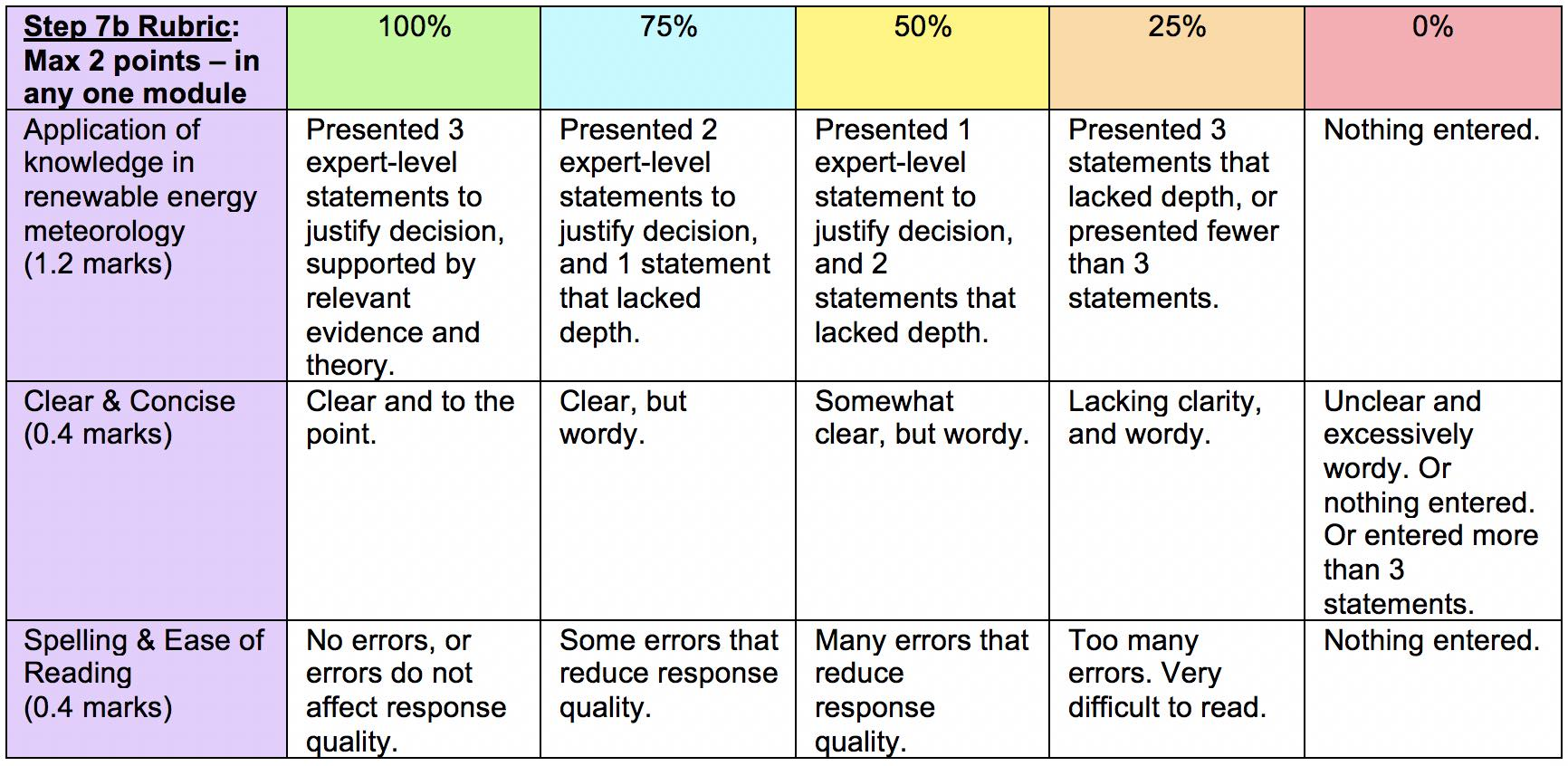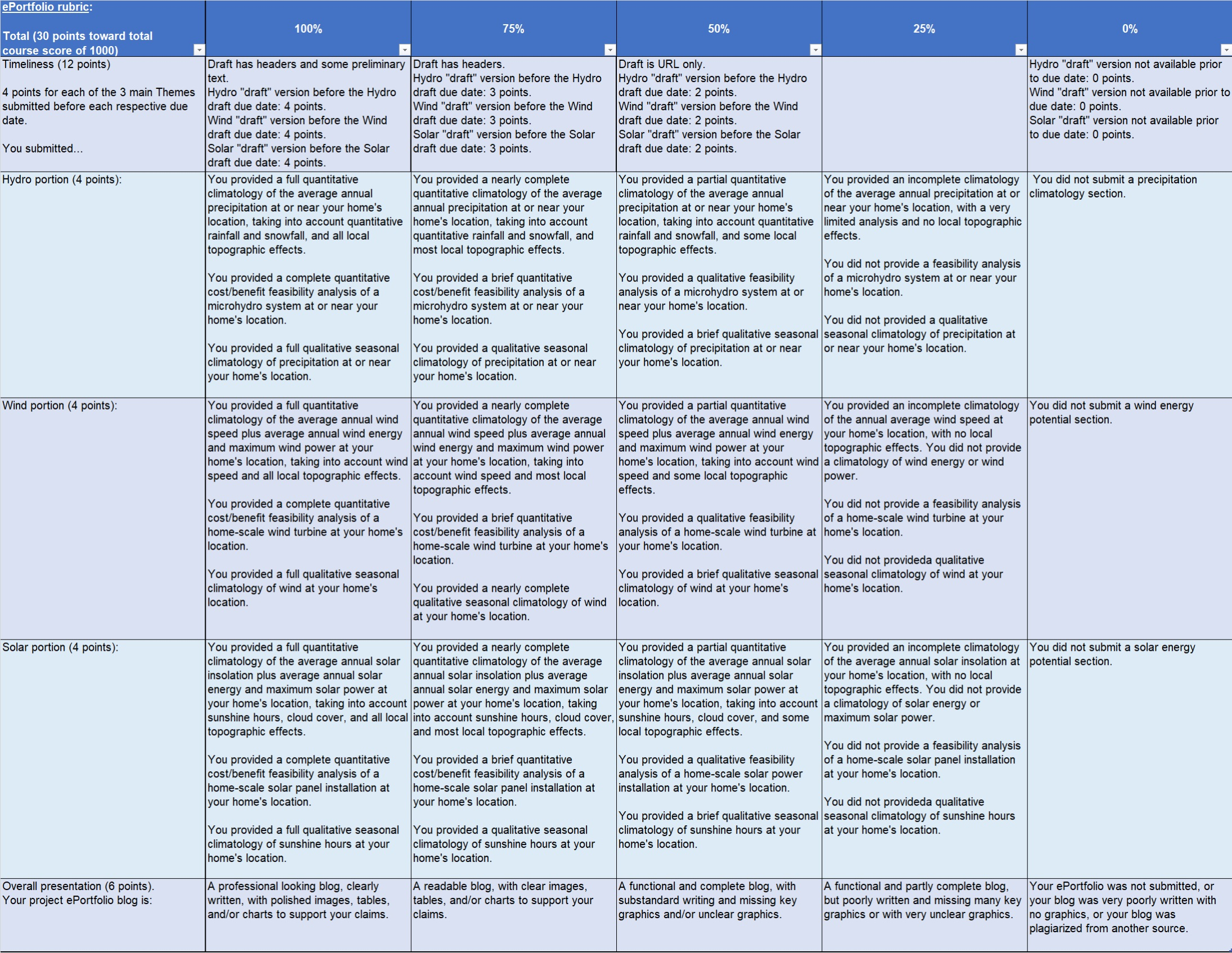UBC ATSC 313 Renewable Energy Meteorology

## Subject: Evaluation

#### Test and assignment weights, grading rubrics, and requirements to pass the course.

39% (= 390/1000 points) case-study modules
(= 3% for each of the 12 "A","B",& "C" modules + 1% for each of the 3 "D" modules)
3% (=   30/1000 points) ePortfolio home renewable energy calculation project.
30% (= 300/1000 points) midterm exams (open book) (= 10% each for 3 exams)
28% (= 280/1000 points) final exam (open book). The final exam consists of an individual portion (24.5% or 245 points) and a group portion (3.5% or 35 points)

3.5% (= 35/1000 points) bonus, for optional end-of-theme and end-of-term surveys
======
100% total (=1035 points truncated to 1000 points).

Each case-study A, B & C module is worth the same amount (= 3% of the overall course grade = 30/1000 points). Within any one module, the relative contributions to the module grade are:

Step 3 questions to ask = 2 points
Step 4 meteorology quizzes = 18 points (total, from all the quizzes)
Step 6 calculations & analysis = 4 points
Step 7a decision = 4 points
Step 7b justification = 2 points
==================
Subtotal = 30 points

As this is an online course , students must have an overall course grade of 50% or better AND an individual final exam grade of 50% or better to pass the course.  See details at the end of this web page.

### Point Diagram

Here are the point diagrams for current, past and future terms.  The point diagrams show how points can be earned during the course.  They are arranged chronologically.

Future terms:

Current term:

Past terms:

• As mentioned before, all multiple-choice quizzes (Module Steps 4, 7a) and midterm exams are automatically marked by Canvas. The final exam is automatically marked (using Canvas or using ReMark software).
• The short-answer of Step 3 (where students write three meteorology questions they want to ask about that case study), the code/analysis submission of Step 6, the justification of Step 7b, and the ePortfolio are hand marked by TAs, according to the following rubrics.

#### Step 3 Rubric

Each question in Step 3 is equally weighted, and is marked using the following key considerations.Each module has a different step 6 assignment. Step 6 assignments are graded according to the thoroughness, completeness and quality of each student's submission.

#### Step 7b Rubric

The grading rubric for question 7b (the justification for your decision on the case study) is:#### ePortfolio Rubric

The ePortfolio project is marked by the instructor & TAs. The ePortfolio has the following key points along a continuous 100% to 0% grading scale.Responses that plagiarize published material or copy answers from other students will earn zero and an invitation to visit the instructor for intervention or to discuss remedial actions.

## Requirements to Pass the Course

To pass the course, you must:

• pass the individual portion of the open-book  final exam (i.e., earn a grade of at least 50%),
AND
• earn an total course score of at least 50%.

The way this is implemented is as follows.